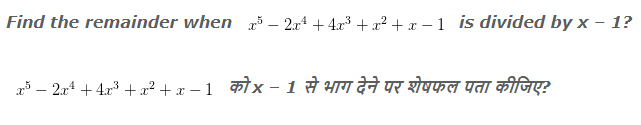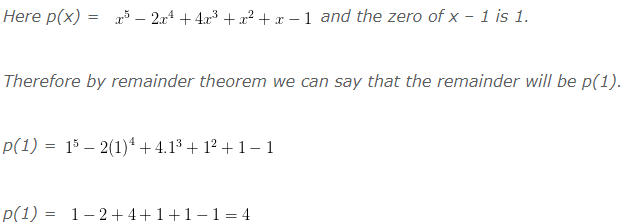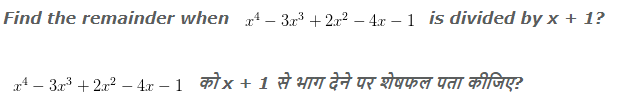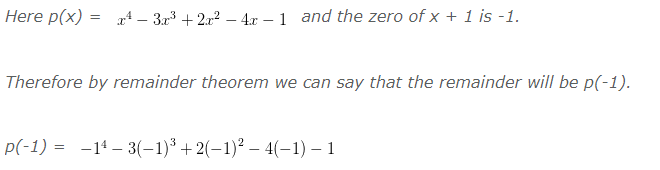# Polynomial Remainder Theorem Examples With Answers

### Remainder Theorem (शेषफल प्रमेय)

Let p(x) be any polynomial of degree greater than or equal to 1 and let ‘a’ be any real number. If p(x) is divided by the linear polynomial (x – a), then the remainder is p(a).

Proof: Let p(x) be any polynomial. Suppose that when p(x) is divided by x – a, then quotient is q(x) and remainder is r(x). i.e.

Since the degree of (x – a) is 1 then the degree of r(x)is less than the degree of x – a, the degree of r(x) = 0. This means that r(x) is a constant, say r.
So for every value of x, r(x) = r.
therefore, p(x) = (x – a)q(x) + r
If x = a, then the equation will give us:
p(a) = (a – a)q(a) + r = 0 + r
p(a) = 0
Which proves the theorem.

### Polynomial Remainder Theorem Examples With Answers

Example 1:-Explanation:So the remainder will be 4.

Example 2:-Explanation:p(-1) = 1+3+2+4-1

So the remainder will be 9.

Example 3:-

Check whether x – 2 is a factor of x³+x²-2x-8

जांच कीजिए कि x – 2, x³+x²-2x-8 का एक गुणानखण्ड है या नहीं?

Explanation:

If x – 2 is a factor of x³+x²-2x-8 then when we will divide x³+x²-2x-8 by x – 2 then remainder must be zero.

So by remainder theorem:

p(x) = x³+x²-2x-8 and the zero of  x – 2 is 2.

So by remainder theorem we can say that the remainder will be p(2).

p(2) = 2³+2²-2(2)-8

= 8+4-4-8

= 0

So there remainder is zero that means x – 2 is a factor of x³+x²-2x-8

### 1 thought on “Polynomial Remainder Theorem Examples With Answers”

1.I was recommended this website by my cousin. I’m not sure whether this post is written by him as nobody else know such detailed about my trouble. You are incredible! Thanks!